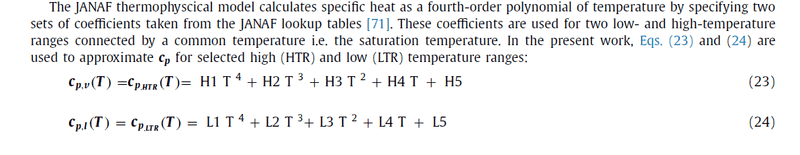# Finding specific heat C_p coefficients using NIST

• JD_PM

#### JD_PM

TL;DR Summary
I am trying to find the specific heat (at constant pressure) ##C_p## coefficients linked to the JANAF model
I am trying to find the specific heat (at constant pressure) ##C_p## coefficients linked to the JANAF model, which basically assumes that ##C_p## is a polynomic function of ##T##, for liquid nitrogen (at ##\approx## 97 K).

Before doing that, I am trying to find those for water (at ##\approx## 300 K) and verify that they are ##9850.69, -48.6714, 0.13736## and ##-0.000127063##.

I am looking into NIST data for water; I checked both gas and condensed thermochemistry data but the coefficients don't match with the above. I also checked the NIST-JANAF thermo tables, for water, but the coefficients are not there.

How to find the desired coefficients for water (at ##\approx## 300 K)? They should be somewhere in the NIST-JANAF table.

Once I see the above it should be straightforward to find those for liquid nitrogen

Thank you!PS: Actually, I am implementing the thermo-physical properties of liquid nitrogen.

Before doing that, I am trying to find those for water (at ##\approx## 300 K) and verify that they are ##9850.69, -48.6714, 0.13736## and ##-0.000127063##.
Where do these come from ? How do they lead to a ##\ c_p=75.349\ ## J/(K.mol) as expected ?

##\ ##

•JD_PM
Just for the record, here's an explanation of the JANAF modelWhere do these come from ? How do they lead to a ##\ c_p=75.349\ ## J/(K.mol) as expected ?

##\ ##

Good question, here is the derivation.

I am trying to find the coefficients for liquid nitrogen online. However, I think the best I could do is follow the same procedure to derive them (not only for ##c_p## but for the density, dynamic viscosity and thermal conductivity).

So I am going to take the temperature range ##-193 < T < -173## Celsius (using ##80K < T < 100K## should also work).

First I am trying to find the density, ##c_p##, dynamic viscosity and thermal conductivity data associated to this temperature range (as done for water in the above derivation).

Good question, here is the derivation.

Still think there is a mismatch somewhere. I can find no way to get a reasonable ##c_p## with e.g. formula ##(23)## or ##(24)## with such coefficients $$9850.69*300^4 -48.6714*300^3+ 0.13736*300^2 -0.000127063*300 = 7.979 \times 10^{13}\ \ ?$$

##\ ##

I am looking into NIST data for water; I checked both gas and condensed thermochemistry data but the coefficients don't match with the above.
The NIST data is for the Shomate equation, which is not polynomial (note the ##E/t^2## term).

•JD_PM
Still think there is a mismatch somewhere. I can find no way to get a reasonable ##c_p## with e.g. formula ##(23)## or ##(24)## with such coefficients $$9850.69*300^4 -48.6714*300^3+ 0.13736*300^2 -0.000127063*300 = 7.979 \times 10^{13}\ \ ?$$

##\ ##

There are no formulas numbered 23 nor 24. Did you mean 7.4 here?

Just for the record, here's an explanation of the JANAF model
https://www.physicsforums.com/attachments/298450